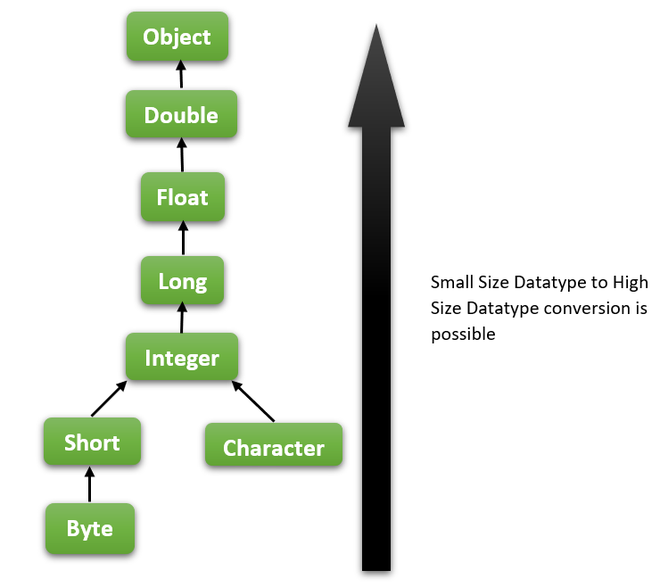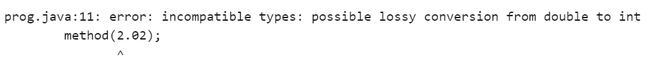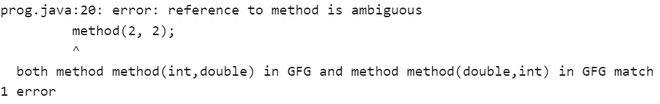Open in App
Not now

• Last Updated : 11 Jan, 2022

Before going into the actual topic, first, we need to know about method overloading and type promotions.

When a class consists of more than one method with the same name but with different signatures and return types, then we call those overloaded methods, and the process is called method overloading.

Example:

```void method(int i)
int method(int i,int j)
void method(double d)```

#### What is Automatic Type Promotion?

The name Type Promotion specifies that a small size datatype can be promoted to a large size datatype. i.e., an Integer data type can be promoted to long, float, double, etc. This Automatic Type Promotion is done when any method which accepts a higher size data type argument is called with the smaller data type.

Example:

```public void method(double a){
System.out.println("Method called");
}

public static void main(){
method(2);
}```

In the above method call, we passed an integer as an argument, but no method accepts an integer in the below code. The Java compiler won’t throw an error because of the Automatic Type Promotion. The Integer is promoted to the available large size datatype, double.

Note:- This is important to remember is Automatic Type Promotion is only possible from small size datatype to higher size datatype but not from higher size to smaller size. i.e., integer to character is not possible.Type Promotion Hierarchy

Example 1: In this example, we are testing the automatic type promotion from small size datatype to high size datatype.

## Java

 `class` `GFG {` `  `  `    ``// A method that accept double as parameter` `    ``public` `static` `void` `method(``double` `d)` `    ``{` `        ``System.out.println(` `            ``"Automatic Type Promoted to Double-"` `+ d);` `    ``}` `  `  `    ``public` `static` `void` `main(String[] args)` `    ``{` `        ``// method call with int as parameter` `        ``method(``2``);` `    ``}` `}`

Output

`Automatic Type Promoted to Double-2.0`

Explanation: Here we passed an Integer as a parameter to a method and there is a method in the same class that accepts double as parameter but not Integer. In this case, the Java compiler performs automatic type promotion from int to double and calls the method.

Example 2: Let’s try to write a code to check whether the automatic type promotion happens from high size datatype to small size datatype.

## Java

 `class` `GFG {` `  `  `    ``// A method that accept integer as parameter` `    ``public` `static` `void` `method(``int` `i)` `    ``{` `        ``System.out.println(` `            ``"Automatic Type Promoted possible from high to small?"``);` `    ``}` `  `  `    ``public` `static` `void` `main(String[] args)` `    ``{` `        ``// method call with double as parameter` `        ``method(``2.02``);` `    ``}` `}`

Output:Error message

Explanation: From this example, it is proven that Automatic Type Promotion is only applicable from small size datatype to big size datatype. As the double size is large when compared to integer so large size to small size conversion fails.

Example 3: In this example, we are going to look at the overloaded methods and how the automatic type of promotion is happening there.

## Java

 `class` `GFG {` `  `  `    ``// A method that accept integer as parameter` `    ``public` `static` `void` `method(``int` `i)` `    ``{` `        ``System.out.println(` `            ``"Automatic Type Promoted to Integer-"` `+ i);` `    ``}` `  `  `    ``// A method that accept double as parameter` `    ``public` `static` `void` `method(``double` `d)` `    ``{` `        ``System.out.println(` `            ``"Automatic Type Promoted to Double-"` `+ d);` `    ``}` `  `  `    ``// A method that accept object as parameter` `    ``public` `static` `void` `method(Object o)` `    ``{` `        ``System.out.println(``"Object method called"``);` `    ``}` `  `  `    ``public` `static` `void` `main(String[] args)` `    ``{` `        ``// method call with char as parameter` `        ``method(``'a'``);` `      `  `        ``// method call with int as parameter` `        ``method(``2``);` `      `  `        ``// method call with float as parameter` `        ``method(``2``.0f);` `      `  `        ``// method call with a string as parameter` `        ``method(``"Geeks for Geeks"``);` `    ``}` `}`

Output

```Automatic Type Promoted to Integer-97
Automatic Type Promoted to Integer-2
Automatic Type Promoted to Double-2.0
Object method called```

Explanation: In the above code,

• First, we called a method with a character as a parameter but we didn’t have any method that is defined that accepts character so it will check the next high size datatype, i.e., Integer. If there is a method that accepts Integer, then it performs automatic type promotion and call that method. If not found, it searches for the next level higher size datatype.
• Here we have a method that accepts Integer so character ‘a’ is converted to an integer- 97, and that respective method is called.
• Next, we called a method by passing two integer variables. As it directly found a method, no promotions happened, and the method is called.
• Next, a float variable is passed, i.e., 2.0f. Here we have a method that accepts double, so the float is converted to double and the method is called.
• Finally, a string is passed which occupies more space when compared to double. So its searches for the methods that accept either a string or Object which is a superclass of all types. In this code, there is no method that accepts string but there is a method that accepts objects. So that method is called after type promotion.

Example 4: In this example, consider the overloaded methods with more than one argument and observe how automatic type conversion is happening here:

## Java

 `class` `GFG {` `  `  `    ``// overloaded methods` `    ``// Method that accepts integer and double` `    ``public` `static` `void` `method(``int` `i, ``double` `d)` `    ``{` `        ``System.out.println(``"Integer-Double"``);` `    ``}` `    ``// Method that accepts double and integer` `    ``public` `static` `void` `method(``double` `d, ``int` `i)` `    ``{` `        ``System.out.println(``"Double-Integer"``);` `    ``}` `    ``public` `static` `void` `main(String[] args)` `    ``{` `        ``// method call by passing integer and double` `        ``method(``2``, ``2.0``);` `        ``// method call by passing double and integer` `        ``method(``2.0``, ``2``);` `        ``// method call by passing both integers` `        ``// method(2, 2);` `        ``// Ambiguous error` `    ``}` `}`

Output

```Integer-Double
Double-Integer```

Explanation: In the above code, when we pass arguments to a method call, the compiler will search for the corresponding method that accepts the same arguments. If present, then it will call that method. Else, it will look for scenarios for automatic type promotion.

• For the first method call, there is already a method that accepts similar arguments, so that it will call that Integer-Double method.
• For the second method call also there is a method defined in the class, and the compiler will call the respective method. (Double-Integer)
• But when we pass 2 Integers as arguments, the Compiler first checks for a respective method that accepts 2 integers. In this case, there is no method that accepts two integers. So it will check for scenarios for type promotion.
• Here there are 2 methods that accept an integer and double and any of the integers can be promoted to double simply, but the problem is ambiguity. The compiler didn’t know what method to call if the type was promoted. So compiler throws an error message like specified below if we uncomment line 20 in the above code-Ambiguity Error

These are the few examples that can give clear insight on Automatic type conversion in overloaded methods.

My Personal Notes arrow_drop_up
Related Articles